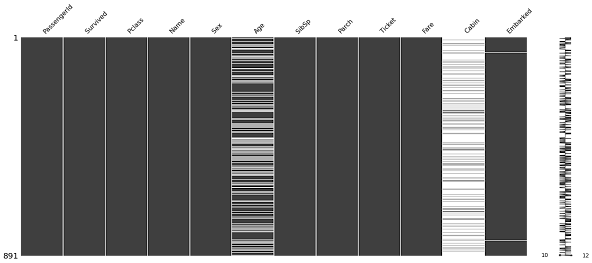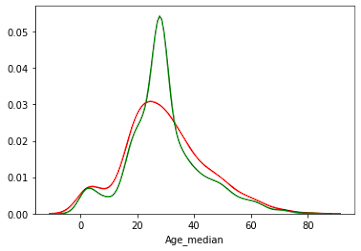# Master important techniques to Handle Missing Values in Machine Learning | Feature Engineering Part-I

Hello, Most of the beginners or machine learning practitioners are stuck in search of the best imputation technique for missing values. As exploring and preparing the data is a crucial task, It needs to be done very carefully by using the right technique in the right situation is necessary.

In this tutorial, we will see various missing value imputation techniques along with their pros and cons and which technique to use in which case. The objective is to learn these techniques is to understand the impact of variable and machine learning models. Let's start with a small introduction to missing values and how they get a place in our datasets.The dataset we will be using is a very popular Titanic dataset for applying each technique. You can easily download the dataset from Kaggle and without downloading also you can create a Kaggle notebook and practice all the techniques.

### What are Missing Values

The field which is left unfilled with the values is referred to as missing data. Sometimes missing data is also marked as not available(NA), not a number(NAN), unknown, etc. There are various reasons for this kind of field remaining unfilled or the researcher or the person who is gathering the data left some fields with empty data.

### Reasons for Presence of Missing Data

1. Manual Error:- Most of the time data we got for analysis is from various surveys and in that form it's not compulsory to fill all the fields so some of the fields are left unfilled by people.
2. Equipment Error:- Also known as instrument error. measurements taken from instruments sometimes left the filled empty.
3. Web scrapping:- In today's scenarios web scrapping tools and techniques are used to extract out the data through websites and during that process due to any computation error missing data takes place.
4. IoT Devices:- IoT sensors are also used for data gathering which also causes the missing values.

### Problems of Missing Data

1. Loss of Efficiency:- Missing data need to be handled before modeling otherwise there can be errors and performance will not seem efficient
2. Complications in handling and analyzing the data
3. bias resulting from a difference between missing and complete data

### Types of Missing Data

Missing data can be classified into 3 categories. Let us explore each type of missing data.

#### 1) Missing Completely as Random(MCAR)

It means there is no relationship between missing data and observed data. It can be easily understood in the dataset that the missing values which we have do not depend on any row or column.

#### 2) Missing at Random(MAR)

It means there is a systematic relationship between the missing values. for example that after every 2 observations we have 1 missing observation then these types of missing data are referred to as missing at random.

#### 3) Missing Not at random(MNAR)

It is a little bit complicated to figure it out and deal with it. The fact behind these missing values is related to unobserved data. for example, the fields that relate to some factors that we did not account for.

### How to Visualize Missing Data using Python

If we have a very huge dataset then using Matplotlib or seaborn does not seem a good choice for missing data visualization so in that case, we have a library known as missingno in python which can be used for missing data imputation.

So moving to a code environment let us code to plot a chart to visualize missing data. We are using the Titanic dataset and I hope you have downloaded it till now.We can see from the above-plotted graph that the age and cabin column contains a missing value and 2-3 missing values are also there in embarked column.

## Various Techniques For Missing Data Imputation

Now we know how to visualize missing values and why it's important to handle them. let's start studying each technique used for Missing Data Imputation. we will be seeing the missing value imputation technique according to Numerical and Categorical variables and also the technique that is used in both the variables.

### Missing value imputation techniques for Numerical Variables

#### 1) Mean-Median Imputation

The technique consists of imputing missing values with the mean or median of all the observations. Some points related mean-median imputation technique that you should remember.

• When the variable has normal distribution then the mean and median are approximately the same.
• Whenever there is skewed distribution, we use the median which is the best representation.
• This technique can be used in deployment if you have missing values of less than 5 percent.

let us try this technique for the age variable and visualize distribution concerning imputation and without imputation.

Code -We can see that after imputation(green line) seems more normally distributed.

Benefits of Mean-Median Imputation
1. Easy to implement
2. can be used in production(Deployment process)
3. It is a fast way of obtaining the complete dataset without writing much code.
Disadvantages of Mean Median Imputation
1. It distorts the distribution of the original variable
2. The higher the percentage of missing values, the higher is a distortion

#### 2) Arbitrary Value Imputation

In this technique, we manually assign a value to all missing values towards an edge means which is out of boundary towards negative or positive.

Code:

Benefits of Arbitrary value Imputation
1. Easy to implement
2. If some fields are not mandatory then can be used in the production
3. Captures the importance of missing values
Disadvantages of Arbitrary Value Imputation
1. Distorts the distribution and variance of a variable
2. If the arbitrary value is at the end then It may create outliers.

#### 3) End Of Distributions

It is almost similar to arbitrary value imputation but the arbitrary value is selected utilizing formula and distribution.

• If the distribution is normal, we can use mean plus/minus the 3 times of standard distribution
• If the distribution is skewed then we use the IQR proximity rule.
Normal Distribution:
mean ± 3 * Standard_distribution

Skewed Distribution:
IQR = 75th Quantile - 25th Quantile
Upper Limit = 75th Quantile + IQR * 3
Lower Limit = 25th Quantile - IQR * 3

This technique is very much powerful to handle the outliers and is mostly used for missing data imputation in numerical variables.

Now we had completed the missing data imputation techniques for numerical variables. There are some more that we will discuss further in this article. First, let us cover the imputation techniques for categorical variables.

### For Categorical Variables

#### 1) Frequent Category Imputation

The technique is also referred to as Mode Imputation. It simply implies input with a value that occurs the most in all the observations. It is the most used imputation technique for category imputation. Let's apply this technique to the embarked column, as it has fewer missing values.

Code-

Benefits of Frequent Category Imputation
1. Easy to implement
2. The fastest way to obtain the complete datasets
3. Can be used in deployment procedure
Disadvantages of Frequent Category Imputation
1. If there are more missing values, then It can lead to over-representation of the frequent category.
2. Over-representation can also create a rare category in data.
3. It distorts the relationship of the frequent label with other categories in observations

#### 2) Add a Missing Indicator to NAN

In this technique, we try to provide special importance to missing values. It is similar to filling with an arbitrary value.

Code-

1. It creates extra feature space
2. when there are fewer missing values then we cannot use this technique

#### 3) Replacing NAN with a new Category

The technique is very similar to the above one. In this, we add a missing term as an unknown, missing, or anything as we want.

Code-

1. There is not any kind of change seen in a dataset or variable relation because importance is provided to missing values.

### Techniques for Numerical & Categorical Both

Now we have seen the techniques for both numerical and categorical variables. Now let's have a look at the technique that can be applied to both the variables

### 1) Random Sample Imputation

In this technique, we take the sample observation from the available data according to the number of missing values we have and with the help of the index, we randomly impute the missing values with sample observations.

The technique is widely used in category imputation to prevent over-representation of a particular category.

Code:

Advantages of Random Sample Imputation
1. Easy to implement and fast way to get a complete dataset
2. It preserves the variance
3. It can be used in Production
Disadvantages of Random Sample Imputation
1. It can affect the relationship between original categories and imputed categories.

## Wrapping Up

We have learned many techniques to impute missing values. I hope you were able to get all the techniques and implement this in your projects. If the right technique is not used then we end up with inappropriate results. If you have any queries then you can post them in the comment section below.

Keep Learning, Happy Learning
Thank You